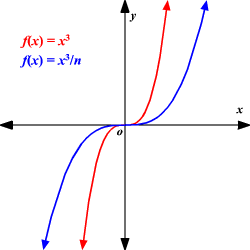# Transformations of Functions

If you start with a simple parent function $y=f\left(x\right)$ and its graph, certain modifications of the function will result in easily predictable changes to the graph.

For example:

Horizontal Shift

• Replacing $f\left(x\right)$ with $f\left(x-b\right)$ results in the graph being shifted $b$ units to the right.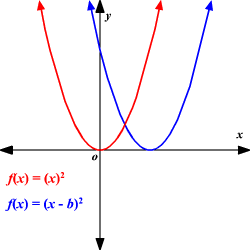\

• Replacing $f\left(x\right)$ with $f\left(x+b\right)$ results in the graph being shifted $b$ units to the left.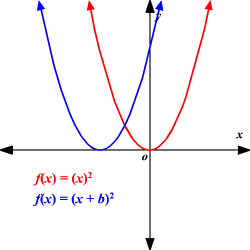Vertical Shift

• Replacing $f\left(x\right)$ with $f\left(x\right)+c$ results in the graph being shifted $c$ units up.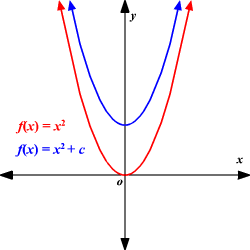• Replacing $f\left(x\right)$ with $f\left(x\right)-c$ results in the graph being shifted $c$ units down.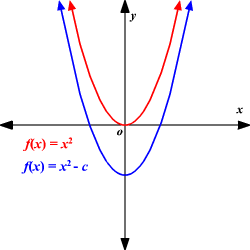Reflection

• Replacing $x$ with $-x$ results in the graph being reflected across the $y$ -axis.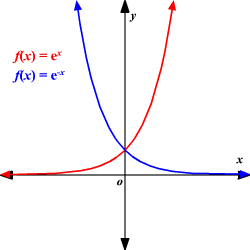• Replacing $f\left(x\right)$ with $-f\left(x\right)$ results in the graph being reflected across the $x$ -axis.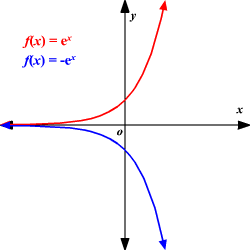Horizontal Stretch/Compression

• Replacing $x$ with $nx$ results in a horizontal compression by a factor of $n$ .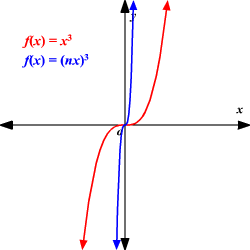• Replacing $x$ with $\frac{x}{n}$ results in a horizontal stretch by a factor of $n$ .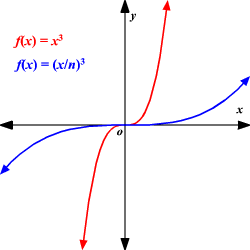Vertical Stretch/Compression

• Replacing $f\left(x\right)$ with $nf\left(x\right)$ results in a vertical stretch by a factor of $n$ .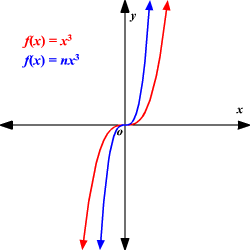• Replacing $f\left(x\right)$ with $\frac{f\left(x\right)}{n}$ results in a vertical compression by a factor of $n$ .